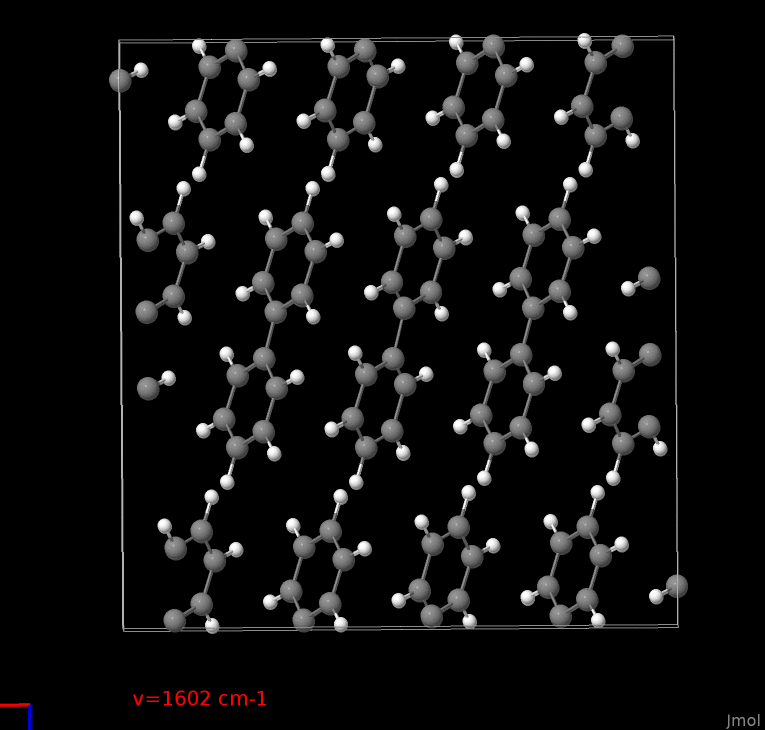PHY.K02UF Molecular and Solid State Physics

## Phonon Modes

In a normal mode, all of the atoms oscillate with the same frequency. The following two modes are of the organic crystal of biphenyl. The positions of the atoms and the symmetries are specified in biphenyl_TS.cifThis is an optical phonon mode of a crystal of biphenyl molecules at $$k=0$$ with a frequency of 1602 cm-1 = 48.03 THz.This is another optical phonon mode of a crystal of biphenyl molecules at $$k=0$$ with a frequency of 65 cm-1 = 1.949 THz.

The following three modes are of a Metal Organic Framework (MOF). The positions of the atoms and the symmetries are specified in MOF.cifThis is an optical phonon mode of a MOF at $$k=0$$ with a frequency of 35.46 cm-1 = 1.063 THz.This is an optical phonon mode of a MOF at $$k=0$$ with a frequency of 21.85 cm-1 = 0.655 THz.This is an optical phonon mode of a MOF at $$k=0$$ with a frequency of 34.21 cm-1 = 1.026 THz.

The phonon modes were calculated with VASP and Phonopy by Natalia Bedoya and Tomas Kamencek. They were animated using JMOL.

Below is a phonon mode at the W point of the Brillouin zone of a GaAS crystal. Press play to start.

The GaAs video was made by Lukas Legenstein.